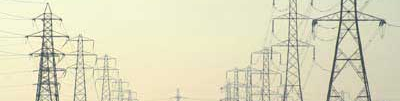# Power Factor Correction

## What is power factor?

Power factor is the ratio of the KW to the KVA of the load and is a measure of how efficiently the current is being used. If the power factor is less than 1.0, the current is not being used to maximum efficiency.

## What causes a bad power factor?

There are two types or sources of reduced power factor, Displacement Power Factor and Distortion Power Factor.

## What is displacement power factor?

Displacement Power Factor is caused by a reactive component in the load. If there is an inductive compinent in the load, then there will be an inductive current flowing in addition to the resistive current. The inductive curent follows the voltage waveform by 90 degrees. Likewaise a capacitive component causes a capacitive current that leads the voltage waveform by 90 degrees. The vector sum of the reactive (capacitive and/or inductive) current(s) and the resistive current results in a single current with a phase angle before (leading) or after(lagging) the voltage waveform. The displacement power factor value is the cosine of the angle between the voltage waveform and the resultant current waveform.
Displacement power factor is typically decreased (made worse) by inductive loads such as induction motors, transformers and lighting ballasts.

## What is distortion power factor?

Distortion power factor is caused by non sinusoidal load currents which are made up of harmonic currents. The harmonic currents are caused by solid state rectifiers and controllers.
Poor distortion power factor is commonly caused by VFDs (Variable Frequency Drives), DC Drives, switch mode power supplies, computors and most electronic products.

## What are the benefits of power factor correction

• Reduced Energy Costs. (reduced KVA charges)
• Lower transmission and distribution losses.
• Better voltage regulation.
• Increased Supply Capacity.
• Reduced non productive load on system.
• Reduced Supply Current

## Industrial Power Factor Correction

Power Factor comes in two flavours, displacement and distortion.
Displacement Power Factor is caused by inductive loads such as induction motors and can be corrected by adding power factor corection capacitors.
Distortion Power Factor is caused by non linear loads such as switchmode power supplies and VFDs that cause harmonic currents. Distortion pPower Factor can only be corrected by the use of either active or passive filters.

## Domestic Power Factor Correction

There are no domestic penalties in New Zealand for having a poor power factor, so there is no advantage in adding power factor correction in the average domestic installation.

## Power Factor Correction Paper

Free download of our power factor correction paper covering displacment power factor and distortion power factor.

New Zealand residents only

- Limited time introductory offer

- register NOW or miss out

- While stocks last